## How to Calculate and Solve for Horizontal Strain | Rock Mechanics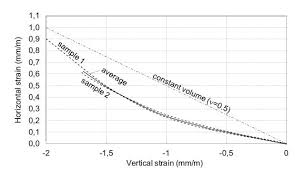The image above represents horizontal strain.

To compute for horizontal strain, five essential parameters are needed and these parameters are Principal Horizontal Stress Component 1 (σH1), Principal Horizontal Stress Component 2 (σH2), Poisson’s Ratio (v), Vertical Stress (σv) and Young’s Modulus (E).

The formula for calculating the horizontal strain:

εH = H1 – vσH2 – vσv) / E

Where:

εH = Horizontal Strain
σH1 = Principal Horizontal Stress Component 1
σH2 = Principal Horizontal Stress Component 2
v = Poisson’s Ratio
σv = Vertical Stress
E = Young’s Modulus

Let’s solve an example;
Find the horizontal strain when the principal horizontal stress component 1 is 7, the principal horizontal component 2 is 9, the Poisson’s ratio is 11, the vertical stress is 21 and young’s modulus is 27.

This implies that;

σH1 = Principal Horizontal Stress Component 1 = 7
σH2 = Principal Horizontal Stress Component 2 = 9
v = Poisson’s Ratio = 11
σv = Vertical Stress = 21
E = Young’s Modulus = 27

εH = H1 – vσH2 – vσv) / E
εH = (7 – 11(9) – 11(21)) / 27
εH = (7 – 99 – 231) / 27
εH = -323 / 27
εH = -11.96

Therefore, the horizontal strain is -11.96.

Calculating the Principal Horizontal Stress Component 1 when the Horizontal Stress, Principal Horizontal Stress Component 2, Poisson’s Ratio, Vertical Stress and Young’s Modulus is Given.

σH1 = (εH x E) + vσH2 + vσv

Where;

σH1 = Principal Horizontal Stress Component 1
εH = Horizontal Strain
σH2 = Principal Horizontal Stress Component 2
v = Poisson’s Ratio
σv = Vertical Stress
E = Young’s Modulus

Let’s solve an example;
Find the principal horizontal stress component 1 when the horizontal strain is 22, the principal horizontal component 2 is 10, the Poisson’s ratio is 7, the vertical stress is 12 and young’s modulus is 3.

This implies that;

εH = Horizontal Strain = 22
σH2 = Principal Horizontal Stress Component 2 = 10
v = Poisson’s Ratio = 7
σv = Vertical Stress = 12
E = Young’s Modulus = 3

σH1 = (εH x E) + vσH2 + vσv
σH1 = (22 x 3) + 7(10) + 7(12)
σH1 = 66 + 70 + 84
σH1 = 220

Therefore, the principal horizontal stress component 1 is 220.

Calculating the Principal Horizontal Stress Component 2 when the Horizontal Stress, Principal Horizontal Stress Component 1, Poisson’s Ratio, Vertical Stress and Young’s Modulus is Given.

σH2 = -(H x E) – σH1 + vσv / v)

Where;

σH2 = Principal Horizontal Stress Component 2
εH = Horizontal Strain
σH1 = Principal Horizontal Stress Component 1
v = Poisson’s Ratio
σv = Vertical Stress
E = Young’s Modulus

Let’s solve an example;
Find the principal horizontal stress component 2 when the horizontal strain is 28, the principal horizontal component 1 is 14, the Poisson’s ratio is 5, the vertical stress is 17 and young’s modulus is 7.

This implies that;

εH = Horizontal Strain =28
σH1 = Principal Horizontal Stress Component 1 = 14
v = Poisson’s Ratio = 5
σv = Vertical Stress = 17
E = Young’s Modulus = 7

σH2 = -(H x E) – σH1 + vσv / v)
σH2 = -((28 x 7) – 14 + 5(17) / 5)
σH2 = -(196 – 14 + 85 / 5)
σH2 = -(182 + 85 / 5)
σH2 = -(267 / 5)
σH2 = -(53.4)
σH2 = -53.4

Therefore, the principal horizontal stress component 2 is -53.4.

## How to Calculate and Solve for Poisson’s Ratio | Rock Mechanics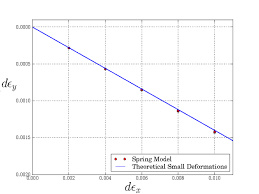The image above represents Poisson’s ratio.

To compute for Poisson’s ratio, two essential parameters are needed and these parameters are lateral strain (εland axial strain (εa).

The formula for calculating Poisson’s ratio:

v = εl / εa

Where:

v = Poisson’s Ratio
εl = Lateral Strain
εa = Axial Strain

Let’s solve an example;
Find the Poisson’s ratio when the lateral strain is 80 and the axial strain is 4.

This implies that;

εl = Lateral Strain = 80
εa = Axial Strain = 4

v = εl / εa
v = 80 / 4
v = 20

Therefore, the Poisson’s ratio is 20.

Calculating the Lateral Strain when the Poisson’s Ratio and Axial Strain is Given.

εl = v x εa

Where:

εl = Lateral Strain
v = Poisson’s Ratio
εa = Axial Strain

Let’s solve an example;
Find the lateral strain when the Poisson’s ratio is 40 and the axial strain is 9.

This implies that;

v = Poisson’s Ratio = 40
εa = Axial Strain = 9

εl = v x εa
εl = 40 x 9
εl = 360

Therefore, the lateral strain is 360.

## How to Calculate and Solve for Young’s Modulus | Rock Mechanics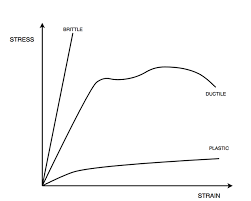The image above represents young’s modulus.

To compute for young’s modulus, two essential parameters are needed and these parameters are axial stress (σa) and axial strain (εa).

The formula for calculating the young’s modulus:

E = σa / εa

Where:

E = Young’s Modulus
σa = Axial Stress
εa = Axial Strain

Let’s solve an example;
Given that the axial stress is 65 and the axial strain is 45. Calculate for the young’s modulus?

This implies that;

σa = Axial Stress = 65
εa = Axial Strain = 45

E = σa / εa
E = 65 / 45
E = 1.44

Therefore, the young’s modulus is 1.44.

Calculating the Axial Stress when the Young’s Modulus and the Axial Strain is Given.

σa = E x εa

Where;

σa = Axial Stress
E = Young’s Modulus
εa = Axial Strain

Let’s solve an example;
Given that the young’s modulus is 25 and the axial strain is 10. Calculate for the axial stress?

This implies that;

E = Young’s Modulus = 25
εa = Axial Strain = 10

σa = E x εa
σa = 25 x 10
σa = 250

Therefore, the axial stress is 250.

## How to Calculate and Solve for Vertical Strain | Rock Mechanics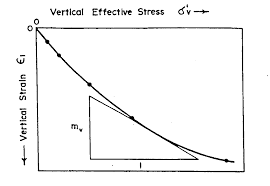The image above represents vertical strain.

To compute for vertical strain, five essential parameters are needed and these parameters are Vertical Stress (σv), Poisson’s Ratio (v), Principal Horizontal Stress Component 1 (σH1), Principal Horizontal Stress Component 2 (σH2) and Young’s Modulus (E).

The formula for calculating the vertical strain:

εv = v – vσH1 – vσH2) / E

Where:

εv = Vertical Strain
σv = Vertical Stress
v = Poisson’s Ratio
σH1 = Principal Horizontal Stress Component 1
σH2 = Principal Horizontal Stress Component 2
E = Young’s Modulus

Let’s solve an example;
Find the vertical strain with a vertical stress of 44, Poisson’s ratio of 5, principal horizontal stress component 1 of 6, principal horizontal stress component 2 of 4 and young’s modulus of 12.

This implies that;

σv = Vertical Stress = 44
v = Poisson’s Ratio = 5
σH1 = Principal Horizontal Stress Component 1 = 6
σH2 = Principal Horizontal Stress Component 2 = 4
E = Young’s Modulus = 12

εv = v – vσH1 – vσH2) / E
εv = (44 – 5(6) – 5(4)) / 12
εv = (44 – 30 – 20) / 12
εv = -6 / 12
εv = -0.5

Therefore, the vertical strain is -0.5.

Calculating the Vertical Stress when the Vertical Strain, Poisson’s Ratio, Principal Horizontal Stress Component 1, Principal Horizontal Stress Component 2 and Young Modulus is Given.

σv = (εv x E) + vσH1 + vσH2

Where:

σv = Vertical Stress
εv = Vertical Strain
v = Poisson’s Ratio
σH1 = Principal Horizontal Stress Component 1
σH2 = Principal Horizontal Stress Component 2
E = Young’s Modulus

Let’s solve an example;
Find the vertical stress with a vertical strain of 52, Poisson’s ratio of 12, principal horizontal stress component 1 of 15, principal horizontal stress component 2 of 10 and young’s modulus of 13.

This implies that;

εv = Vertical Strain = 52
v = Poisson’s Ratio = 12
σH1 = Principal Horizontal Stress Component 1 = 15
σH2 = Principal Horizontal Stress Component 2 = 10
E = Young’s Modulus = 13

σv = (εv x E) + vσH1 + vσH2
σv = (52 x 13) + 12(15) + 15(10)
σv = 676 + 180 + 150
σv = 1006

Therefore, the vertical stress is 1006.

Calculating the Poisson’s Ratio when the Vertical Strain, Vertical Stress, Principal Horizontal Stress Component 1, Principal Horizontal Stress Component 2 and Young Modulus is Given.

v = σv – (εv x E) / σH1 + σH2

Where:

v = Poisson’s Ratio
εv = Vertical Strain
σv = Vertical Stress
σH1 = Principal Horizontal Stress Component 1
σH2 = Principal Horizontal Stress Component 2
E = Young’s Modulus

Let’s solve an example;
Find the Poisson’s ratio with a vertical strain of 16, vertical stress of 110, principal horizontal stress component 1 of 7, principal horizontal stress component 2 of 13 and young’s modulus of 6.

This implies that;

εv = Vertical Strain = 16
σv = Vertical Stress = 110
σH1 = Principal Horizontal Stress Component 1 = 7
σH2 = Principal Horizontal Stress Component 2 = 13
E = Young’s Modulus = 6

v = σv – (εv x E) / σH1 + σH2
v = 110 – (16 x 6) / 7 + 13
v = 110 – 96 / 20
v = 14 / 20
v = 0.7

Therefore, the Poisson’s ratio is 0.7.

## How to Calculate and Solve for Horizontal Stress | Rock Mechanics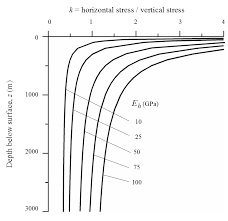The image above represents horizontal stress.

To compute for horizontal stress, two essential parameters are needed and these parameters are Poisson’s ratio (v) and vertical stress (σv).

The formula for calculating the horizontal stress:

σH = v / 1 – v

Where;

σH = Horizontal Stress
v = Poisson’s Ratio
σv = Vertical Stress

Let’s solve an example;
Find the horizontal stress when the Poisson’s ratio is 24 and the vertical stress is 28.

This implies that;

v = Poisson’s Ratio = 24
σv = Vertical Stress = 28

σH = v / 1 – v
σH = 24(28) / (1 – 24)
σH = 672 / -23
σH = -29.217

Therefore, the horizontal stress is 29.217.

Calculating the Poisson’s Ratio when the Horizontal Stress and Vertical stress is Given.

v = σH / σv + σH

Where;

v = Poisson’s Ratio
σH = Horizontal Stress
σv = Vertical Stress

Let’s solve an example;
Find the Poisson’s ratio when the horizontal stress is 12 and the vertical stress is 16.

This implies that;

σH = Horizontal Stress = 12
σv = Vertical Stress = 16

v = σH / σv + σH
v = 12 / 16 + 12
v = 12 / 28
v = 12 / 28
v = 0.428

Therefore, the Poisson’s ratio is 0.428.

## How to Calculate and Solve for Lateral Strain | Rock Mechanics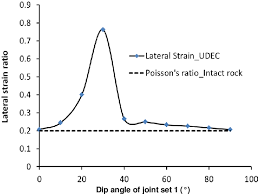The image above represents lateral strain.

To compute for lateral strain, two essential parameters are needed and these parameters are change in diameter (Δd) and diameter (d).

The formula for calculating the lateral strain:

εl = Δd / d

Where:

εl = Lateral Strain
Δd = Change in Diameter
d = Diameter

Let’s solve an example;
Find the lateral strain when the change in diameter is 32 and the diameter is 14.

This implies that;

Δd = Change in Diameter = 32
d = Diameter = 14

εl = Δd / d
εl = 32 / 14
εl = 2.285

Therefore, the lateral strain is 2.285.

Calculating the Change in Diameter when the Lateral Strain and the Diameter is Given.

Δd = εl  x d

Where;

Δd = Change in Diameter
εl = Lateral Strain
d = Diameter

Let’s solve an example;
Find the change in diameter when the lateral strain is 40 and the diameter is 8.

This implies that;

εl = Lateral Strain = 40
d = Diameter = 8

Δd = εl  x d
Δd = 40 x 8
Δd = 320

Therefore, the change in diameter is 320.

## How to Calculate and Solve for Axial Strain | Rock Mechanics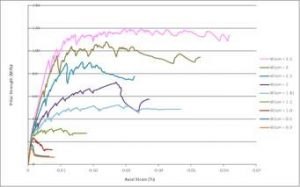The image above represents axial strain.

To compute for axial strain, two essential parameters are needed and these parameters are change in length (ΔL) and length (L).

The formula for calculating the axial strain:

εo = ΔL / L

Where;

εo = Axial Strain
ΔL = Change in Length
L = Total Length

Let’s solve an example;
Calculate the axial strain when the change in length is 18 and total length is 32.

This implies that;

ΔL = Change in Length = 18
L = Total Length = 32

εo = ΔL / L
εo = 18 / 32
εo = 0.5625

Therefore, the axial strain is 0.5625.

Calculating the Change in Length when the Axial Strain and Total Length is Given.

ΔL = εo x L

Where;

ΔL = Change in Length
εo = Axial Strain
L = Total Length

Let’s solve an example;
Calculate the change in length when the axial strain is 21 and total length is 9

This implies that;

εo = Axial Strain = 21
L = Total Length = 9

ΔL = εo x L
ΔL = 21 x 9
ΔL = 189

Therefore, the change in length is 189.

## How to calculate and Solve for Shear Stress | Rock Mechanics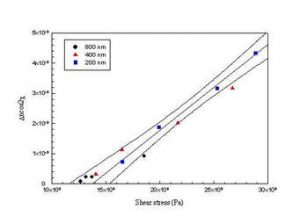The image above represents shear stress.

To compute for shear stress, two essential parameters are needed and these parameters are Shear Force (ΔS) and Area (ΔA).

The formula for calculating the shear stress:

τ = ΔS / ΔA

Where:

τ = Shear Stress
ΔS = Shear Force
ΔA = Area

Let’s solve an example;
Find the shear stress when the shear force is 16 with an area of 30.

This implies that;

ΔS = Shear Force = 16
ΔA = Area = 30

τ = ΔS / ΔA
τ = 16 / 30
τ = 0.53

Therefore, the shear stress is 0.53 Pa.

Calculating the Shear Force when the Shear Stress and Area is Given.

ΔS = τ x ΔA

Where;

ΔS = Shear Force
τ = Shear Stress
ΔA = Area

Let’s solve an example;
Find the shear force with a shear stress of 40 and an area of 12.

This implies that;

τ = Shear Stress = 40
ΔA = Area = 12

ΔS = τ x ΔA
ΔS = 40  x 12
ΔS = 480

Therefore, the Shear Force is 480.

## How to Calculate and Solve for Normal Stress | Rock Mechanics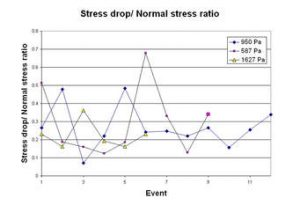The image above represents normal stress.

To calculate for normal stress, two essential parameters are needed and these parameters are normal force (ΔN) and area (ΔA).

The formula for calculating the normal stress:

σn = ΔN / ΔA

Where;

σn = Normal Stress
ΔN = Normal Force
ΔA = Area

Let’s solve an example;
Calculate the normal stress with a normal force of 12 and an area of 22.

This implies that;

ΔN = Normal Force = 12
ΔA = Area = 22

σn = ΔN / ΔA
σn = 12 / 22
σn = 0.54

Therefore, the normal stress is 0.54 Pa.

Calculating the Normal Force when the Normal Stress and Area is Given.

ΔN = σn x ΔA

Where;

ΔN = Normal Force
σn = Normal Stress
ΔA = Area

Let’s solve an example;
Calculate the normal force when the normal stress is 25 with an area of 10.

This implies that;

σn = Normal Stress = 25
ΔA = Area = 10

ΔN = σn x ΔA
ΔN = 25 x 10
ΔN = 250

Therefore, the normal force is 250.